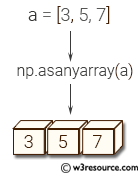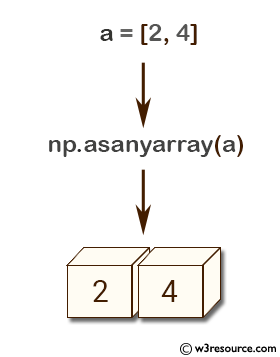# NumPy array creation: asanyarray() function

## asanyarray() function

The asanyarray() function is used to convert the input to an ndarray, but pass ndarray subclasses through.

Syntax:

`numpy.asanyarray(a, dtype=None, order=None)`Version: 1.15.0

Parameter:

Name Description Required /
Optional
a Input data, in any form that can be converted to an array. This includes scalars, lists, lists of tuples, tuples, tuples of tuples, tuples of lists, and ndarrays. Required
dtype By default, the data-type is inferred from the input data. optional
order Whether to use row-major (C-style) or column-major (Fortran-style) memory representation. Defaults to 'C'. optional

Return value:

[ndarray]
Array interpretation of a. No copy is performed if the input is already an ndarray with matching dtype and order. If a is a subclass of ndarray, a base class ndarray is returned.

Example-1: NumPy.asanyarray() function

``````>>> import numpy as np
>>> a = [2, 4]
>>> np.asanyarray(a)
array([2, 4])
``````

Pictorial Presentation:Example-2: NumPy.asanyarray() function

``````>>> import numpy as np
>>> a = np.array([(2.0, 3), (3.0, 5)], dtype='f4,i4').view(np.recarray)
>>> np.asanyarray(a) is a
True
``````

Python - NumPy Code Editor:

Previous: asarray()
Next: ascontiguousarray()

﻿